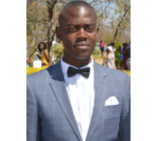# Kumbirai K - Tutor in Kempton Park Cbd

I am an Engineer by profession, I am very good in mathematics and science. I have 3 years post graduate experience in Engineering. I do part time tutoring for N3 to Btech level for mathematics and engineering science.

###### Teaching places

My Home, Students Home, Public Place, and Online

###### Travelling distance

Kumbirai will travel 60km from Kempton Park Cbd, Kempton Park, Gauteng, South Africa

###### Languages spoken

Kumbirai speaks English

###### Experience
2016-08-01 - 2019-05-29
###### Mechanical Engineer

I specialise in design and supervision of installation of various mechanical systems

###### Education
2011-03-01 - 2015-04-17

###### Subjects taught
• Physical Science

I did mathematics from matric to university level. At matric i was very good in mathematics and science. At university level i did advanced engineering mathematics for the first two years which involve pure mathematics, trigonometry, statistics and linear algebra. We also did Engineering science which include- mechanics, fluid dynamics, solid mechanics, design thermodynamics

Kumbirai teaches Physical Science at University/College level(s)

• Mechanical Engineering

I did mathematics from matric to university level. At matric i was very good in mathematics and science. At university level i did advanced engineering mathematics for the first two years which involve pure mathematics, trigonometry, statistics and linear algebra. We also did Engineering science which include- mechanics, fluid dynamics, solid mechanics, design thermodynamics

Kumbirai teaches Mechanical Engineering at University/College level(s)

• Calculus

I did mathematics from matric to university level. At matric i was very good in mathematics and science. At university level i did advanced engineering mathematics for the first two years which involve pure mathematics, trigonometry, statistics and linear algebra

Kumbirai teaches Calculus at University/College level(s)

• Mathematics

I did mathematics from matric to university level. At matric i was very good in mathematics and science. At university level i did advanced engineering mathematics for the first two years which involve pure mathematics, trigonometry, statistics and linear algebra

Kumbirai teaches Mathematics at University/College level(s)

• Algebra

I did mathematics from matric to university level. At matric i was very good in mathematics and science. At university level i did advanced engineering mathematics for the first two years which involve pure mathematics, trigonometry, statistics and linear algebra

Kumbirai teaches Algebra at University/College level(s)

• Linear Algebra

I did mathematics from matric to university level. At matric i was very good in mathematics and science. At university level i did advanced engineering mathematics for the first two years which involve pure mathematics, trigonometry, statistics and linear algebra

Kumbirai teaches Linear Algebra at University/College level(s)

• Trigonometry

I did mathematics from matric to university level. At matric i was very good in mathematics and science. At university level i did advanced engineering mathematics for the first two years which involve pure mathematics, trigonometry, statistics

Kumbirai teaches Trigonometry at University/College level(s)